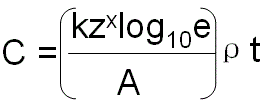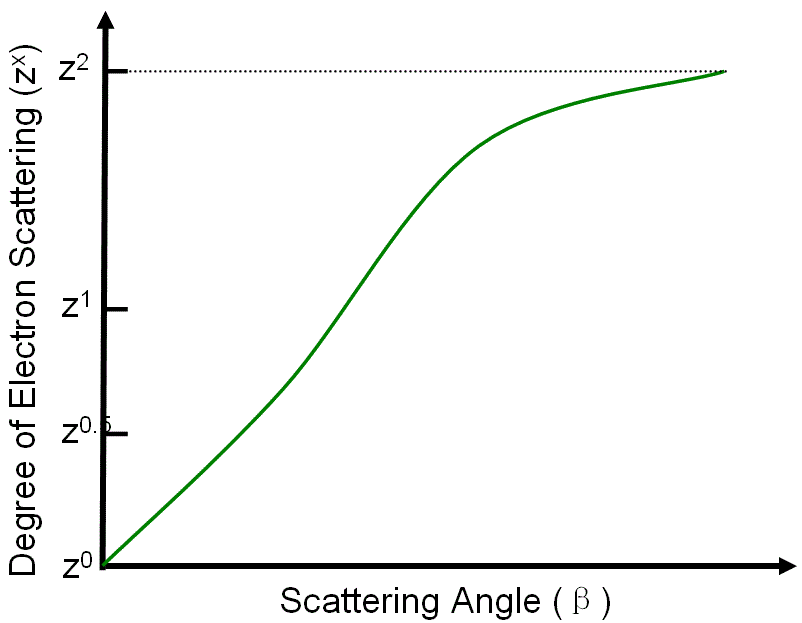Mass-Thickness Contrast in STEM Images
- Practical Electron Microscopy and Database -
- An Online Book -
Microanalysis | EM Book                                                                                   http://www.globalsino.com/EM/

=================================================================================

STEM image contrast C can be given by (see page1226),
C = ln(1/T) -------------------------------------------------------------------------------- [1225a]
= nσx ------------------------------------------------------------------------------------ [1225b]
= n(σe + σi)t ---------------------------------------------------------------------------- [1225c]
where,
σ -- The total cross section for scattering.
σe -- The cross section for elastic scattering.
σi -- The cross section for inelastic scattering.
t -- The thickness of the specimen.
T -- The transmission of incident electrons through a substance.

Since the scattering cross section σ relates to the atomic number Z with a power-law dependence Zx, a local contrast in STEM images can also be written in the empirical form below,------------------------------------------------------ [1225d]-------------------------- [1225e]--------- [1225f]
where,
k and x -- The parameters that vary depending on the incident electron energy and collection semiangle.
e -- A numerical constant ( 2.71828).
A -- The atomic weight of the element.
ρ -- The density of the specimen.

Figure 1225 shows the atomic number (zx) dependence on scattering angle β at high voltages. At large scattering angle, electron scattering is proportional to Z2 according to the Rutherford approximation but the exponent falls to zero with the decrease of scattering angle. If ρ/A is equal for all the elements in the TEM specimen, then the contrast depends only on zx (e.g. Z contrast in HAADF STEM imaging).Figure 1225. Atomic number (zx) dependence on scattering angle β at high voltages.

Since k·log10e is a material-independent factor, then the local contrast depends on the mass and thickness of the specimen as shown in Equation [1225e], and thus it is called mass-thickness contrast.

Note that the relations described in Equations 1225d - 1225f will break down for thick specimens above a certain thickness, due to multiple scattering of electrons, which depends on the specimen material, accelerating voltage and collection semi-angle.

Table 1225. Thickness dependence of validation of Equations 1225d - 1225f.

Specimen thickness
Validation of Equations 1225d - 1225f
Thick
Not valid
Thin
Valid

=================================================================================